# JP5073601B2 - Battery state estimation method and power supply device - Google Patents

## Info

Publication number
JP5073601B2
JP5073601B2 JP2008180687A JP2008180687A JP5073601B2 JP 5073601 B2 JP5073601 B2 JP 5073601B2 JP 2008180687 A JP2008180687 A JP 2008180687A JP 2008180687 A JP2008180687 A JP 2008180687A JP 5073601 B2 JP5073601 B2 JP 5073601B2
Authority
JP
Japan
Prior art keywords
battery
value
voltage value
function
kalman filter
Prior art date
Legal status (The legal status is an assumption and is not a legal conclusion. Google has not performed a legal analysis and makes no representation as to the accuracy of the status listed.)
Expired - Fee Related
Application number
JP2008180687A
Other languages
Japanese (ja)
Other versions
JP2010019705A (en
Inventor

Original Assignee

Priority date (The priority date is an assumption and is not a legal conclusion. Google has not performed a legal analysis and makes no representation as to the accuracy of the date listed.)
Filing date
Publication date
Application filed by 住友電気工業株式会社, 住友電装株式会社, 株式会社オートネットワーク技術研究所 filed Critical 住友電気工業株式会社
Priority to JP2008180687A priority Critical patent/JP5073601B2/en
Publication of JP2010019705A publication Critical patent/JP2010019705A/en
Application granted granted Critical
Publication of JP5073601B2 publication Critical patent/JP5073601B2/en
Expired - Fee Related legal-status Critical Current
Anticipated expiration legal-status Critical

• 238000005070 sampling Methods 0.000 claims description 14
• 239000011159 matrix materials Substances 0.000 description 13
• 230000000875 corresponding Effects 0.000 description 5
• 230000002123 temporal effects Effects 0.000 description 5
• 239000003570 air Substances 0.000 description 2
• 238000010586 diagrams Methods 0.000 description 2
• 230000003068 static Effects 0.000 description 2
• 238000007476 Maximum Likelihood Methods 0.000 description 1
• 238000004364 calculation methods Methods 0.000 description 1
• 239000003897 fog Substances 0.000 description 1

## Images

••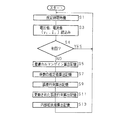•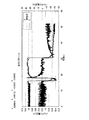•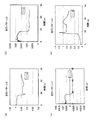•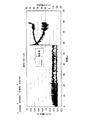••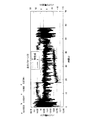•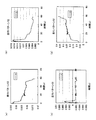•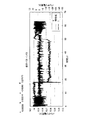•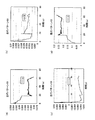•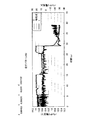•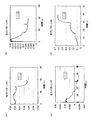•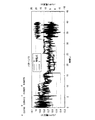•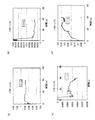## Classifications

• YGENERAL TAGGING OF NEW TECHNOLOGICAL DEVELOPMENTS; GENERAL TAGGING OF CROSS-SECTIONAL TECHNOLOGIES SPANNING OVER SEVERAL SECTIONS OF THE IPC; TECHNICAL SUBJECTS COVERED BY FORMER USPC CROSS-REFERENCE ART COLLECTIONS [XRACs] AND DIGESTS
• Y02TECHNOLOGIES OR APPLICATIONS FOR MITIGATION OR ADAPTATION AGAINST CLIMATE CHANGE
• Y02EREDUCTION OF GREENHOUSE GAS [GHG] EMISSIONS, RELATED TO ENERGY GENERATION, TRANSMISSION OR DISTRIBUTION
• Y02E60/00Enabling technologies; Technologies with a potential or indirect contribution to GHG emissions mitigation
• Y02E60/10Energy storage using batteries

## Description

In Patent Document 1, a plurality of voltage / current value pairs are sampled during a cranking period during the start-up period of the vehicle engine, a regression line is obtained from the collected voltage / current value pairs, and an internal value is obtained from the slope. There is disclosed a battery state quantity computing device that calculates a resistance value, obtains a pseudo open voltage value, calculates a difference between pseudo open voltage values before and after engine start, and corrects an internal resistance value based on the difference. In Patent Document 1, a plurality of voltage / current value pairs are sampled during a cranking period during the start-up period of the vehicle engine, a regression line is obtained from the collected voltage / current value pairs, and an internal value is obtained from the slope. There is disclosed a battery state quantity computing device that calculates a resistance value, obtains a pseudo open voltage value, calculates a difference between pseudo open voltage values ​​before and after engine start, and corrects an internal resistance value based on the difference ..
JP 2007-223530 A JP 2007-223530 A

The present invention has been made in view of the above-described circumstances, and an object of the first invention is to provide a battery state estimation method capable of knowing battery characteristics in consideration of temporal variation. . The present invention has been made in view of the above-described circumstances, and an object of the first invention is to provide a battery state estimation method capable of knowing battery characteristics in consideration of temporal variation.
It is an object of the second invention to provide a power supply apparatus capable of knowing battery characteristics in consideration of temporal variations. It is an object of the second invention to provide a power supply apparatus capable of knowing battery characteristics in consideration of temporal variations.

この電源装置が内部抵抗値を推定する対象は車両用のバッテリ２である。 The target for which this power supply device estimates the internal resistance value is the vehicle battery 2. バッテリ２及びオルタネータ（車載発電機、交流発電機）３は、電気負荷であるエアコン（エアコンディショナ）／ヒータ１０、ヘッドライト１１、フォグランプ１２及びデフォガ１３等に、イグニッションスイッチ７（ここでは、アクセサリスイッチも含むものとする）を通じて電力を供給する。 The battery 2 and the alternator (vehicle-mounted generator, alternator) 3 are electric loads such as an air conditioner (air conditioner) / heater 10, a headlight 11, a fog lamp 12, a defogger 13, and an ignition switch 7 (here, an accessory). Power is supplied through the switch). Hereinafter, the present invention will be described with reference to the drawings illustrating embodiments thereof. Embodied, the present invention will be described with reference to the drawings illustrating embodiments thereof.
FIG. 1 is a block diagram showing a schematic configuration of an embodiment of a battery state estimation method and a power supply device according to the present invention. FIG. 1 is a block diagram showing a schematic configuration of an embodiment of a battery state estimation method and a power supply device according to the present invention.
The object for which the power supply device estimates the internal resistance value is a vehicle battery 2. A battery 2 and an alternator (on-vehicle generator, AC generator) 3 are connected to an air conditioner (air conditioner) / heater 10, which is an electric load, a headlight 11, a fog lamp 12, a defogger 13, and the like, and an ignition switch 7 (here, an accessory) (Including switches). The object for which the power supply device estimates the internal resistance value is a vehicle battery 2. A battery 2 and an alternator (on-vehicle generator, AC generator) 3 are connected to an air conditioner (air conditioner) / heater 10, which is an electric load, a headlight 11, a fog lamp 12, a defogger 13, and the like, and an ignition switch 7 (here, an accessory) (Including switches).

バッテリ２は、例えば鉛蓄電池であって、一方の端子はイグニッションスイッチ７に接続され、他方の端子は接地されている。オルタネータ３は、車両のエンジン４に連動して、バッテリ２を適宜充電する。
バッテリ状態推定部１は、マイクロコンピュータで構成されており、電圧検出部６が検出したバッテリ２の両極間の電圧値Ｖ、及び電流検出部５が検出したバッテリ２を流れる電流値Ｉが与えられ、時系列的にサンプリングする。 The battery state estimation unit 1 is composed of a microcomputer, and is given a voltage value V between both poles of the battery 2 detected by the voltage detection unit 6 and a current value I flowing through the battery 2 detected by the current detection unit 5. , Sampling in chronological order.
バッテリ状態推定部１が推定した内部抵抗値は、バッテリ２の残容量の指標として表示部９に表示される。 The internal resistance value estimated by the battery state estimation unit 1 is displayed on the display unit 9 as an index of the remaining capacity of the battery 2. The battery 2 is, for example, a lead storage battery, and one terminal is connected to the ignition switch 7 and the other terminal is grounded. The alternator 3 charges the battery 2 as appropriate in conjunction with the engine 4 of the vehicle. The alternator 3 charges the battery 2 as appropriate in conjunction with the engine 4 of the vehicle. The battery 2 is, for example, a lead storage battery, and one terminal is connected to the ignition switch 7 and the other terminal is grounded.
The battery state estimation unit 1 is configured by a microcomputer, and is given a voltage value V between both electrodes of the battery 2 detected by the voltage detection unit 6 and a current value I flowing through the battery 2 detected by the current detection unit 5. Sampling in time series. The battery state estimation unit 1 is configured by a microcomputer, and is given a voltage value V between both electrodes of the battery 2 detected by the voltage detection unit 6 and a current value I flowing through the battery 2 detected by the current detection unit 5 . Sampling in time series.
The internal resistance value estimated by the battery state estimation unit 1 is displayed on the display unit 9 as an indicator of the remaining capacity of the battery 2. The internal resistance value estimated by the battery state estimation unit 1 is displayed on the display unit 9 as an indicator of the remaining capacity of the battery 2.

バッテリ状態推定部１は、記憶部１ａを備えており、記憶部１ａは、バッテリ２の電圧値及び電流値の相前後する２回のサンプリングの内、前の電圧値及び電流値と後の電流値とをそれぞれ変数とし、後の電圧値を表す関数（バッテリの特性式）を記憶している。この関数は、例えば式（１）のように表される。
t ＝ａＶ t-1 ＋ｂＩ t ＋ｃＩ t-1 ＋ｄ （１） V t = aV t-1 + bI t + cI t-1 + d (1)

V t = aV t-1 + bI t + cI t-1 + d (1) V t = aV t-1 + bI t + cI t-1 + d (1)
Note that the two samplings do not need to be adjacent in chronological order, and include, for example, a mode in which they are moved one sampling period at a time separated by three sampling periods. Note that the two samplings do not need to be adjacent in chronological order, and include, for example, a mode in which they are moved one sampling period at a time separated by three sampling periods.

また、式（１）の係数から下記により内部抵抗値を求められることが分かっている。

Further, it has been found that the internal resistance value can be obtained from the coefficient of the equation (1) by the following.
Dynamic internal resistance value = b (when current flows)
Static internal resistance value = -c / a (when no current flows)

t ＝（Ｖt-1 ，Ｉt ，Ｉt-1，１） （２）
t ＝（ａ，ｂ，ｃ，ｄ）T （３）
（Ｔ；転置行列）
The storage unit 1a also stores a Kalman filter created based on the function shown in Expression (1).
The function shown in Expression (1) can be expressed as shown in Expression (4) by multiplication of the matrix H t shown in Expression (2) and the matrix x t shown in Expression (3).
H t = (V t−1 , I t , I t−1 , 1) (2) H t = (V t−1 , I t , I t−1 , 1) (2)
x t = (a, b, c, d) T x t = (a, b, c, d) T (3) (3)
(T: transposed matrix) (T: transposed matrix)

ここで、観測量（検出したバッテリ２の電圧値Ｖ t ）をｙ tとすると、記憶部１ａが記憶しているカルマンフィルタの観測方程式は、式（５）で示される。
t ＝Ｈ tt ＋ｖ t （５）

y t = H t x t + v t (5) y t = H t x t + v t (5)
V t shown in Equation (5) is noise according to the covariance matrix R and the zero-mean Gaussian distribution. R represents a matrix that changes with time. V t shown in Equation (5) is noise according to the covariance matrix R and the zero-mean Gaussian distribution. R represents a matrix that changes with time.

このカルマンフィルタの、時点ｔにおける状態ｘ tを１サンプリング前の時点ｔ−１の状態ｘ t-1により表現する状態方程式は、式（６）で示される。
t ＝Ｆｘ t-1 ＋Ｇｗ t （６） x t = Fx t-1 + Gw t (6)

x t = Fx t-1 + Gw t (6) x t = Fx t-1 + Gw t (6)
W t shown in Expression (6) is noise according to the covariance matrix Q and the zero-mean Gaussian distribution, and F, G, and Q indicate matrices that change with time. W t shown in Expression (6) is noise according to the covariance matrix Q and the zero-mean Gaussian distribution, and F, G, and Q indicate matrices that change with time.

ここで、式（３）のｘt が収束するとき、ｘt ，ｘt-1の各最尤値は等しく、Ｇ＝０であるので、式（６）からＦは式（７）に示すような単位行列になる。 Here, when x t in equation (3) converges, the maximum likelihood values of x t and x t−1 are equal and G = 0, so equations (6) through F are expressed in equation (7). It becomes such a unit matrix.

このカルマンフィルタの初期条件は、状態ｘ tについては式（８）のように設定され、誤差の共分散行列Σ t|t-1については式（９）のように設定されている。 The initial conditions of the Kalman filter are set as shown in Equation (8) for the state x t and as shown in Equation (9) for the error covariance matrix Σ t | t−1 .

バッテリ状態推定部１は、先ず、所定時間（例えば数百ミリ秒間）待機した（Ｓ１）後、電圧検出部６で検出したバッテリ２の電圧値Ｖ（＝観測値ｙ t ）と、電流検出部５で検出したバッテリ２を流れる電流値Ｉ（Ｉ t ）とを読込む（Ｓ３）。 The battery state estimation unit 1 first waits for a predetermined time (for example, several hundred milliseconds) (S1), and then the voltage value V (= observed value y t ) of the battery 2 detected by the voltage detection unit 6 and the current detection unit 1. 5 current flowing through the battery 2 detected by the I (I t) and loads the (S3). 次いで、このサンプリング（Ｓ３）が初回であるなら（Ｓ４）、再度、所定時間待機した（Ｓ１）後、バッテリ２の電圧値Ｖ（＝観測値ｙ t ）と電流値Ｉ（Ｉ t ）とを読込む（Ｓ３）。 Then, if the sampling (S3) is the first time (S4), again after waiting a predetermined time (S1), the voltage value of the battery 2 V (= observed value y t) and the current value I and (I t) Read (S3). Hereinafter, the operation of the battery state estimation unit 1 of the power supply apparatus having such a configuration will be described with reference to the flowchart of FIG. Efficiently, the operation of the battery state estimation unit 1 of the power supply apparatus having such a configuration will be described with reference to the flowchart of FIG.
Battery state estimating unit 1, first, a predetermined time (example, several hundred milliseconds) after waiting (S1), the voltage value of the battery 2 detected by the voltage detection unit 6 V (= observed value y t), the current detection unit The current value I (I t ) flowing through the battery 2 detected in 5 is read (S3). Next, if this sampling (S3) is the first time (S4), after waiting again for a predetermined time (S1), the voltage value V (= observed value y t ) and current value I (I t ) of the battery 2 are obtained. Read (S3). Battery state estimating unit 1, first, a predetermined time (example, several hundred milliseconds) after waiting (S1), the voltage value of the battery 2 detected by the voltage detection unit 6 V (= observed value y t), the current detection unit The current value I (I t ) flowing through the battery 2 detected in 5 is read (S3). Next, if this sampling (S3) is the first time (S4), after waiting again for a predetermined time (S1), the voltage value V (= observed value y t) and current value I (I t) of the battery 2 are obtained. Read (S3).

バッテリ状態推定部１は、このサンプリング（Ｓ３）が初回でなければ（Ｓ４）、直近の２回のサンプリングの内、前の電圧値Ｖt-1 及び電流値Ｉt-1 と後の電流値Ｉt（ＨＩt-1 ）を使用して、式（１０）により最適カルマンゲインＫt を算出して、記憶部１ａに記憶する（Ｓ５）。 If this sampling (S3) is not the first time (S4), the battery state estimation unit 1 determines that the previous voltage value V t-1, current value I t-1, and the subsequent current value among the two most recent samplings Using I t (HI t−1 ), the optimum Kalman gain K t is calculated by Equation (10) and stored in the storage unit 1a (S5).

バッテリ状態推定部１は、次に、読込んだ観測値ｙt （Ｓ３）、及び算出した最適カルマンゲイン（Ｓ５）を使用して式（１２）を演算し、式（１）に示す関数の各係数ａ，ｂ，ｃ，ｄの推定値を算出して、記憶部１ａに記憶する（Ｓ７）。 Next, the battery state estimation unit 1 calculates Expression (12) using the read observation value y t (S3) and the calculated optimum Kalman gain (S5), and the function of the function shown in Expression (1) is calculated. Estimated values of the coefficients a, b, c, d are calculated and stored in the storage unit 1a (S7).

バッテリ状態推定部１は、次に、算出した最適カルマンゲイン（Ｓ５）を使用して式（１３）を演算し、誤差行列Σt|t を算出して、記憶部１ａに記憶する（Ｓ９）。 Next, the battery state estimation unit 1 calculates Equation (13) using the calculated optimal Kalman gain (S5), calculates the error matrix Σ t | t , and stores it in the storage unit 1a (S9). .

バッテリ状態推定部１は、次に、算出した誤差行列Σt|t （Ｓ９）を使用して式（１４）を演算し、更新された誤差行列Σt+1|tを算出して、記憶部１ａに記憶する（Ｓ１１）。更新された誤差行列Σt+1|tは、次回のサンプリング時に、式（１０）による最適カルマンゲインＫtの算出（Ｓ５）に使用される。

Note that when x t in equation (3) converges, in equation (14), F = F T = 1 and G = G T = 0. Note that when x t in equation (3) converges, in equation (14), F = F T = 1 and G = G T = 0.

バッテリ状態推定部１は、次に、式（１）に示す関数の算出した各係数ａ，ｂ，ｃ，ｄの推定値（Ｓ７）を使用して、動的内部抵抗値＝ｂ、静的内部抵抗値＝−ｃ／ａを算出し、記憶部１ａに記憶する（Ｓ１３）。次いで、所定時間待機して（Ｓ１）、上述した処理（Ｓ３〜１３）を繰返す。   Next, the battery state estimation unit 1 uses the estimated values (S7) of the coefficients a, b, c, and d calculated by the function shown in the equation (1), and the dynamic internal resistance value = b, static The internal resistance value = −c / a is calculated and stored in the storage unit 1a (S13). Next, after waiting for a predetermined time (S1), the above-described processing (S3 to 13) is repeated.

また、バッテリ状態推定部１は、算出した動的内部抵抗値ｂ（Ｓ１３）を使用して、ＣＣＡ（Cold Cranking Ampere）により下記のようにバッテリ２の劣化度（健全度）を判定することができる。ＣＣＡは、バッテリが満充電の状態で−１８℃のとき、放電開始から３０秒後の最終電圧値が７．２Ｖとなるような放電電流値である。

ｒ（−１８）＝ｂ＋ｆ（Ｔ） （Ｔは現状の測定温度） Moreover, the battery state estimation part 1 determines the deterioration degree (health degree) of the battery 2 as follows by CCA (Cold Cranking Ampere) using the calculated dynamic internal resistance value b (S13). it can. CCA is a discharge current value such that when the battery is at −18 ° C. in a fully charged state, the final voltage value 30 seconds after the start of discharge is 7.2V. r (-18) = b + f (T) (T is the current measured temperature) Moreover, the battery state estimation part 1 determines the deterioration degree (health degree) of the battery 2 as follows by CCA (Cold Cranking Ampere) using the calculated dynamic internal resistance value b (S13). It can. CCA is a discharge current value such that when the battery is at -18 ° C. in a fully charged state, the final voltage value 30 seconds after the start of discharge is 7.2V ..
First, the battery state estimation unit 1 converts the dynamic internal resistance value b into a dynamic internal resistance value at −18 ° C. by the following equation. First, the battery state estimation unit 1 converts the dynamic internal resistance value b into a dynamic internal resistance value at −18 ° C. by the following equation.
r (−18) = b + f (T) (T is the current measured temperature) r (-18) = b + f (T) (T is the current measured temperature)

ＣＣＡnow ＝（ａＶnow ＋ｃＩnow＋ｄ−７．２）／ｒ（−１８）
バッテリ状態推定部１は、算出した現状のバッテリ２のＣＣＡ now値と基準のＣＣＡ値との割合ＣＣＡ now ／ＣＣＡを算出し、算出した割合がＣＣＡ now ／ＣＣＡ＞０．７を満たすか否かを判定する。 Battery state estimating unit 1, calculated by calculating the ratio CCA now / CCA and CCA now value and the reference CCA value of the battery 2 of the present situation, the calculated ratio is whether they meet the CCA now /CCA>0.7 To judge. バッテリ状態推定部１は、判定した結果がＣＣＡ now ／ＣＣＡ＞０．７を満たしていれば、バッテリ２の健全度は良好とし、満たしていなければ、バッテリ２への充電を促進する充電制御、使用する負荷を制限する負荷制御等を実行する。 If the result of the determination satisfies CCA now / CCA> 0.7, the battery state estimation unit 1 considers the soundness of the battery 2 to be good, and if not, the charge control that promotes charging of the battery 2. Execute load control, etc. to limit the load to be used. Next, the battery state estimation unit 1 calculates CCA now , which is the CCA value of the current battery 2 (assuming −18 ° C.) by the following equation. Next, the battery state estimation unit 1 calculates CCA now , which is the CCA value of the current battery 2 (assuming −18 ° C.) by the following equation.
CCA now = (aV now + cI now + d-7.2) / r (-18) CCA now = (aV now + cI now + d-7.2) / r (-18)
The battery state estimation unit 1 calculates a ratio CCA now / CCA between the calculated current CCA now value of the battery 2 and the reference CCA value, and whether or not the calculated ratio satisfies CCA now /CCA>0.7. Determine. The battery state estimation unit 1 determines that the soundness of the battery 2 is good if the determined result satisfies CCA now / CCA> 0.7, and if not, charging control that promotes charging of the battery 2; Execute load control to limit the load to be used. The battery state estimation unit 1 calculates a ratio CCA now / CCA between the calculated current CCA now value of the battery 2 and the reference CCA value, and whether or not the calculated ratio satisfies CCA now / CCA> 0.7. Determine. The battery state estimation unit 1 determines that the soundness of the battery 2 is good if the determined result satisfies CCA now / CCA> 0.7, and if not, charging control that promotes charging of the battery 2; Execute load control to limit the load to be used.

また、式（１）の各係数を推定することにより、現在の状態で大電流負荷△Ｉが掛かったときのバッテリ２の電圧値を推定することができる。現在のバッテリ２の電圧値及び電流値をＶ m ，Ｉ mとすれば、大電流負荷時のバッテリ２の推定電圧値は、
n ＝ａＶ m ＋ｂ（Ｉ m ＋△Ｉ）＋ｃＩ m ＋ｄ V n = aV m + b (I m + ΔI) + cI m + d
で算出でき、大電流作動時に負荷を制限する負荷制御に使用することができる。 It can be calculated with, and can be used for load control that limits the load when operating with a large current.
また、バッテリ２の内部抵抗値を推定できれば、既知であるハーネス抵抗等を使用して、バッテリの現状でエンジンを始動させることができるか否かを推定することができる。 Further, if the internal resistance value of the battery 2 can be estimated, it is possible to estimate whether or not the engine can be started in the current state of the battery by using a known harness resistance or the like. Further, by estimating each coefficient of the equation (1), it is possible to estimate the voltage value of the battery 2 when the large current load ΔI is applied in the current state. If the current voltage value and current value of the battery 2 are V m and I m , the estimated voltage value of the battery 2 at the time of a large current load is Further, by estimating each coefficient of the equation (1), it is possible to estimate the voltage value of the battery 2 when the large current load ΔI is applied in the current state. If the current voltage value and current value of the battery 2 are V m and I m, the estimated voltage value of the battery 2 at the time of a large current load is
V n = aV m + b (I m + ΔI) + cI m + d V n = aV m + b (I m + ΔI) + cI m + d
And can be used for load control to limit the load during large current operation. And can be used for load control to limit the load during large current operation.
Moreover, if the internal resistance value of the battery 2 can be estimated, it is possible to estimate whether the engine can be started with the current state of the battery using a known harness resistance or the like. Moreover, if the internal resistance value of the battery 2 can be estimated, it is possible to estimate whether the engine can be started with the current state of the battery using a known harness resistance or the like.

4A, 4B, 4C, and 4D show transitions of the coefficients a, b, c, and d when the Kalman filter is applied to the characteristic equation (1) in the case of the traveling pattern 2 of the vehicle. It is a graph. Each value of a, b, c, and d in FIG. 3 indicates a convergence value after each transition of the coefficients a, b, c, and d. 4A, 4B, 4C, and 4D show transitions of the coefficients a, b, c, and d when the Kalman filter is applied to the characteristic equation (1) in the case of the traveling pattern 2 of the vehicle. It is a graph .Each value of a, b, c, and d in FIG. 3 indicates a convergence value after each transition of the coefficients a, b, c, and d.
According to FIGS. 3 and 4, for example, the coefficients a, b, c and d change corresponding to a sudden change in voltage value and current value in the vicinity of 18 seconds, and by applying the Kalman filter, the characteristic formula (1) It can be seen that the voltage value of can accurately reproduce the voltage value of the battery 2. According to FIGS. 3 and 4, for example, the coefficients a, b, c and d change corresponding to a sudden change in voltage value and current value in the vicinity of 18 seconds, and by applying the Kalman filter, the characteristic formula ( 1) It can be seen that the voltage value of can accurately reproduce the voltage value of the battery 2.

FIGS. 6A, 6B, 6C, and 6D show transitions of the coefficients a, b, c, and d when the Kalman filter is applied to the characteristic equation (1) in the case of the traveling pattern 4 of the vehicle. It is a graph. Each value of a, b, c, and d in FIG. 5 indicates the convergence value after each transition of the coefficients a, b, c, and d. FIGS. 6A, 6B, 6C, and 6D show transitions of the coefficients a, b, c, and d when the Kalman filter is applied to the characteristic equation (1) in the case of the traveling pattern 4 of the vehicle. It is a graph. Each value of a, b, c, and d in FIG. 5 indicates the convergence value after each transition of the coefficients a, b, c, and d.
According to FIGS. 5 and 6, for example, coefficients a, b, c, and d change corresponding to a sudden change in the voltage value and current value in the vicinity of 58 seconds. By applying the Kalman filter, the characteristic formula (1) It can be seen that the voltage value of can accurately reproduce the voltage value of the battery 2. According to FIGS. 5 and 6, for example, coefficients a, b, c, and d change corresponding to a sudden change in the voltage value and current value in the vicinity of 58 seconds. By applying the Kalman filter, the characteristic formula ( 1) It can be seen that the voltage value of can accurately reproduce the voltage value of the battery 2.

8A, 8B, 8C, and 8D show transitions of the coefficients a, b, c, and d when the Kalman filter is applied to the characteristic equation (1) in the case of the traveling pattern 52 of the vehicle. It is a graph. Each value of a, b, c, and d in FIG. 7 indicates a convergence value after each transition of the coefficients a, b, c, and d. 8A, 8B, 8C, and 8D show transitions of the coefficients a, b, c, and d when the Kalman filter is applied to the characteristic equation (1) in the case of the traveling pattern 52 of the vehicle. It is a graph .Each value of a, b, c, and d in FIG. 7 indicates a convergence value after each transition of the coefficients a, b, c, and d.
According to FIGS. 7 and 8, for example, the coefficients a, b, c, and d change corresponding to a sudden change in the voltage value around 18 seconds and a change in the current value around 50 seconds, and by applying the Kalman filter, It can be seen that the voltage value according to the characteristic equation (1) can accurately reproduce the voltage value of the battery 2. According to FIGS. 7 and 8, for example, the coefficients a, b, c, and d change corresponding to a sudden change in the voltage value around 18 seconds and a change in the current value around 50 seconds, and by applying the Kalman filter, It can be seen that the voltage value according to the characteristic equation (1) can accurately reproduce the voltage value of the battery 2.

FIGS. 10A, 10B, 10C, and 10D show transitions of the coefficients a, b, c, and d when the Kalman filter is applied to the characteristic equation (1) in the case of the traveling pattern 53 of the vehicle. It is a graph. Each value of a, b, c, and d in FIG. 9 indicates a convergence value after each transition of the coefficients a, b, c, and d. FIGS. 10A, 10B, 10C, and 10D show transitions of the coefficients a, b, c, and d when the Kalman filter is applied to the characteristic equation (1) in the case of the traveling pattern 53 of the vehicle. It is a graph. Each value of a, b, c, and d in FIG. 9 indicates a convergence value after each transition of the coefficients a, b, c, and d.
According to FIGS. 9 and 10, for example, the coefficients a, b, c, and d change corresponding to a sudden change in voltage value and current value around 18 seconds and a change in current value around 60 seconds, and the Kalman filter is applied. Thus, it can be seen that the voltage value according to the characteristic equation (1) can accurately reproduce the voltage value of the battery 2. According to FIGS. 9 and 10, for example, the coefficients a, b, c, and d change corresponding to a sudden change in voltage value and current value around 18 seconds and a change in current value around 60 seconds, and the Kalman filter is applied. Thus, it can be seen that the voltage value according to the characteristic equation (1) can accurately reproduce the voltage value of the battery 2.

12A, 12B, 12C, and 12D show transitions of the coefficients a, b, c, and d when the Kalman filter is applied to the characteristic equation (1) in the case of the traveling pattern 63 of the vehicle. It is a graph. Each value of a, b, c, and d in FIG. 11 indicates a convergence value after each transition of the coefficients a, b, c, and d. 12A, 12B, 12C, and 12D show transitions of the coefficients a, b, c, and d when the Kalman filter is applied to the characteristic equation (1) in the case of the traveling pattern 63 of the vehicle. It is a graph .Each value of a, b, c, and d in FIG. 11 indicates a convergence value after each transition of the coefficients a, b, c, and d.
11 and 12, for example, the coefficients a, b, c, and d change corresponding to a sudden change in the voltage value near 15 seconds and a change in the voltage value and current value around 28 seconds, and the Kalman filter is applied. Thus, it can be seen that the voltage value according to the characteristic equation (1) can accurately reproduce the voltage value of the battery 2. 11 and 12, for example, the coefficients a, b, c, and d change corresponding to a sudden change in the voltage value near 15 seconds and a change in the voltage value and current value around 28 seconds, and the Kalman filter is applied Thus, it can be seen that the voltage value according to the characteristic equation (1) can accurately reproduce the voltage value of the battery 2.

14A, 14B, 14C, and 14D show transitions of the coefficients a, b, c, and d when the Kalman filter is applied to the characteristic equation (1) in the case of the traveling pattern 72 of the vehicle. It is a graph. Each value of a, b, c, and d in FIG. 13 indicates a convergence value after each transition of the coefficients a, b, c, and d. 14A, 14B, 14C, and 14D show transitions of the coefficients a, b, c, and d when the Kalman filter is applied to the characteristic equation (1) in the case of the traveling pattern 72 of the vehicle. It is a graph .Each value of a, b, c, and d in FIG. 13 indicates a convergence value after each transition of the coefficients a, b, c, and d.
According to FIGS. 13 and 14, for example, the coefficients a, b, c correspond to the sudden change of the current value around 5 seconds, the sudden change of the voltage value around 15 seconds, and the change of the voltage value and current value around 32, 3 seconds. , D change, and it can be seen that by applying the Kalman filter, the voltage value according to the characteristic equation (1) can accurately reproduce the voltage value of the battery 2. According to FIGS. 13 and 14, for example, the coefficients a, b, c correspond to the sudden change of the current value around 5 seconds, the sudden change of the voltage value around 15 seconds, and the change of the voltage value and current value around 32, 3 seconds., D change, and it can be seen that by applying the Kalman filter, the voltage value according to the characteristic equation (1) can accurately reproduce the voltage value of the battery 2.

１ バッテリ状態推定部
１ａ 記憶部
２ バッテリ
３ オルタネータ
５ 電流検出部
６ 電圧検出部
７ イグニッションスイッチ
９ 表示部
DESCRIPTION OF SYMBOLS 1 Battery state estimation part 1a Memory | storage part 2 Battery 3 Alternator 5 Current detection part 6 Voltage detection part 7 Ignition switch 9 Display part

## Claims (2)

1. バッテリの内部抵抗値を、前記バッテリの電流値及び電圧値を時系列的にサンプリングして推定するバッテリの状態推定方法において、
相前後する２回のサンプリングの内、前の電圧値及び電流値と後の電流値とをそれぞれ変数とし、後の電圧値を表す関数を作成し、作成した関数に基づくカルマンフィルタを作成しておき、サンプリングしたバッテリの電圧値及び電流値を前記カルマンフィルタに逐次適用させることにより、前記関数の各係数を逐次推定し、逐次推定された各係数に基づき、前記バッテリの内部抵抗値を逐次推定することを特徴とするバッテリの状態推定方法。 Of the two samplings before and after the phase, the previous voltage value and current value and the later current value are used as variables, a function representing the later voltage value is created, and a Kalman filter based on the created function is created. , The voltage value and the current value of the sampled battery are sequentially applied to the Kalman filter to sequentially estimate each coefficient of the function, and the internal resistance value of the battery is sequentially estimated based on each sequentially estimated coefficient. A method for estimating the state of a battery, which comprises. In the battery state estimation method for estimating the internal resistance value of the battery by sampling the current value and voltage value of the battery in time series, In the battery state estimation method for estimating the internal resistance value of the battery by sampling the current value and voltage value of the battery in time series,
Of the two samplings that precede and follow, the previous voltage value, current value, and subsequent current value are variables, and a function that represents the subsequent voltage value is created, and a Kalman filter based on the created function is created. , By sequentially applying the sampled voltage value and current value of the battery to the Kalman filter, sequentially estimating each coefficient of the function, and sequentially estimating the internal resistance value of the battery based on each sequentially estimated coefficient The battery state estimation method characterized by these. Of the two samplings that precede and follow, the previous voltage value, current value, and subsequent current value are variables, and a function that represents the subsequent voltage value is created, and a Kalman filter based on the created function is created., By Sequentially applying the sampled voltage value and current value of the battery to the Kalman filter, sequentially estimating each coefficient of the function, and sequentially estimating the internal resistance value of the battery based on each sequentially estimated coefficient The battery state estimation method characterized by these.
2. バッテリの内部抵抗値を、前記バッテリの電流値及び電圧値を時系列的にサンプリングして推定する電源装置において、
相前後する２回のサンプリングの内、前の電圧値及び電流値と後の電流値とをそれぞれ変数とし、後の電圧値を表す関数を記憶する手段と、該手段が記憶する関数に基づき作成されたカルマンフィルタを記憶する手段と、サンプリングしたバッテリの電圧値及び電流値を前記カルマンフィルタに逐次適用して、前記関数の各係数を逐次推定する手段と、該手段が逐次推定した各係数により、前記バッテリの内部抵抗値を逐次推定する手段とを備えることを特徴とする電源装置。 Created based on a means for storing a function representing a later voltage value and a function for storing a function in which the previous voltage value and the current value and the subsequent current value are used as variables in the two samplings before and after the phase. The means for storing the Kalman filter, the means for sequentially applying each coefficient of the function by sequentially applying the voltage value and the current value of the sampled battery to the Kalman filter, and the means for sequentially estimating each coefficient of the function, and the above-mentioned A power supply device including a means for sequentially estimating the internal resistance value of a battery. In the power supply device that estimates the internal resistance value of the battery by sampling the current value and voltage value of the battery in time series, In the power supply device that estimates the internal resistance value of the battery by sampling the current value and voltage value of the battery in time series,
Of the two samplings before and after each other, the previous voltage value, the current value, and the subsequent current value are variables, and a function that stores a function that represents the subsequent voltage value is created based on the function that the means stores. Means for storing the obtained Kalman filter, means for sequentially applying the sampled voltage value and current value of the battery to the Kalman filter, sequentially estimating each coefficient of the function, and each coefficient sequentially estimated by the means, A power supply apparatus comprising: means for sequentially estimating an internal resistance value of the battery. Of the two samplings before and after each other, the previous voltage value, the current value, and the subsequent current value are variables, and a function that stores a function that represents the subsequent voltage value is created based on the function that the means stores Means for storing the obtained Kalman filter, means for sequentially applying the sampled voltage value and current value of the battery to the Kalman filter, sequentially estimating each coefficient of the function, and each coefficient sequentially estimated by the means, A power supply apparatus comprising. : means for sequentially estimating an internal resistance value of the battery.
JP2008180687A 2008-07-10 2008-07-10 Battery state estimation method and power supply device Expired - Fee Related JP5073601B2 (en)

## Priority Applications (1)

Application Number Priority Date Filing Date Title
JP2008180687A JP5073601B2 (en) 2008-07-10 2008-07-10 Battery state estimation method and power supply device

## Applications Claiming Priority (1)

Application Number Priority Date Filing Date Title
JP2008180687A JP5073601B2 (en) 2008-07-10 2008-07-10 Battery state estimation method and power supply device

## Publications (2)

Publication Number Publication Date
JP2010019705A JP2010019705A (en) 2010-01-28
JP5073601B2 true JP5073601B2 (en) 2012-11-14

# Family

## Family Applications (1)

Application Number Title Priority Date Filing Date
JP2008180687A Expired - Fee Related JP5073601B2 (en) 2008-07-10 2008-07-10 Battery state estimation method and power supply device

## Country Status (1)

JP (1) JP5073601B2 (en)

## Cited By (1)

* Cited by examiner, † Cited by third party
Publication number Priority date Publication date Assignee Title
CN103713263A (en) * 2012-09-28 2014-04-09 富士通株式会社 State evaluation apparatus, method and program of secondary battery

## Families Citing this family (1)

* Cited by examiner, † Cited by third party
Publication number Priority date Publication date Assignee Title
CN102680795B (en) * 2012-05-29 2014-11-05 哈尔滨工业大学 Real-time on-line estimation method for internal resistance of secondary battery

## Family Cites Families (3)

* Cited by examiner, † Cited by third party
Publication number Priority date Publication date Assignee Title
CA2588334C (en) * 2004-11-29 2011-09-06 Lg Chem, Ltd. Method and system for joint battery state and parameter estimation
US7589532B2 (en) * 2005-08-23 2009-09-15 Lg Chem, Ltd. System and method for estimating a state vector associated with a battery
JP2010002227A (en) * 2008-06-18 2010-01-07 Autonetworks Technologies Ltd Open-circuit voltage estimating method and power supply

## Cited By (1)

* Cited by examiner, † Cited by third party
Publication number Priority date Publication date Assignee Title
CN103713263A (en) * 2012-09-28 2014-04-09 富士通株式会社 State evaluation apparatus, method and program of secondary battery

## Also Published As

Publication number Publication date
JP2010019705A (en) 2010-01-28

## Similar Documents

Publication Publication Date Title
US10267864B2 (en) Battery management system including apparatus for estimating battery state
US9377512B2 (en) Battery state estimator combining electrochemical solid-state concentration model with empirical equivalent-circuit model
US9157966B2 (en) Method and apparatus for online determination of battery state of charge and state of health
EP2667211B1 (en) Device for estimating state of charge of battery
EP2579381B1 (en) System and method for determination of deterioration of lithium ion secondary battery
US8918300B2 (en) Apparatus and method for battery state of charge estimation
JP3714333B2 (en) Secondary battery input / output possible power estimation device
US7339351B2 (en) Method and apparatus for estimating remaining capacity of secondary battery
CN102253342B (en) Battery state estimator using multiple sampling rates
US10308129B2 (en) Real-time battery estimation
KR101399388B1 (en) Apparatus and Method for estimating the life span of battery
JP4414757B2 (en) In-vehicle battery monitoring apparatus and method
EP2432067B1 (en) Battery system, vehicle, and battery mounted device
JP4649101B2 (en) Secondary battery status detection device and status detection method
US9446678B2 (en) Battery model with robustness to cloud-specific communication issues
EP1380849B1 (en) Process for the determination of the available charge quantity of a storage battery and monitoring device
JP5248764B2 (en) Storage element abnormality detection device, storage element abnormality detection method, and abnormality detection program thereof
US8718988B2 (en) Method for determining and/or predicting the maximum power capacity of a battery
JP4866187B2 (en) Battery control device, electric vehicle, and program for causing computer to execute processing for estimating charge state of secondary battery
US6836122B2 (en) Deterioration degree calculating apparatus and deterioration degree calculating method for a battery
JP5442583B2 (en) State detection device for power supply and power supply device
US8552688B2 (en) On-vehicle battery condition estimation device
JP3960241B2 (en) Secondary battery remaining capacity estimation device, secondary battery remaining capacity estimation method, and computer-readable recording medium storing a program for causing a computer to execute processing by the secondary battery remaining capacity estimation method
EP2551687B1 (en) Device for estimating internal state of battery, and method for estimating internal state of battery
JP4638251B2 (en) Battery management device

## Legal Events

Date Code Title Description
A621 Written request for application examination

Free format text: JAPANESE INTERMEDIATE CODE: A621

Effective date: 20110622

A977 Report on retrieval

Free format text: JAPANESE INTERMEDIATE CODE: A971007

Effective date: 20120720

TRDD Decision of grant or rejection written
A01 Written decision to grant a patent or to grant a registration (utility model)

Free format text: JAPANESE INTERMEDIATE CODE: A01

Effective date: 20120807

A01 Written decision to grant a patent or to grant a registration (utility model)

Free format text: JAPANESE INTERMEDIATE CODE: A01

A61 First payment of annual fees (during grant procedure)

Free format text: JAPANESE INTERMEDIATE CODE: A61

Effective date: 20120822

R150 Certificate of patent or registration of utility model

Free format text: JAPANESE INTERMEDIATE CODE: R150

FPAY Renewal fee payment (event date is renewal date of database)

Free format text: PAYMENT UNTIL: 20150831

Year of fee payment: 3

LAPS Cancellation because of no payment of annual fees